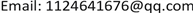Vol. 08  No. 11 ( 2019 ), Article ID: 32893 , 10 pages
10.12677/AAM.2019.811202

Hermite-Hadamard Type Integral Inequality for Coordinated $\left(r,\left(h,m\right)\right)$ -Convex Function

Shuang Gao, Donghai Ji

School of Science, Harbin University of Science and Technology, Harbin HeilongjiangReceived: Oct. 17th, 2019; accepted: Nov. 4th, 2019; published: Nov. 11th, 2019ABSTRACT

Hermite-Hadamard inequality is one of the most important inequalities in convex functions. Its integral error estimates have important applications in optimization and computation. Since the concept of co-convexity of multivariate functions was introduced, the convexity theory has been further developed. In this paper, a new binary function is defined, coordinated $\left(r,\left(h,m\right)\right)$ -convex functions of bivariate functions, one of whose components is r-convex and the other is extended $\left(h,m\right)$ -convex; Hermite-Hadamard type integral inequalities are studied.

Keywords:r-convex, co-ordinated (r,(h,m))-Conve, Hermite-Hadamard Type InequalitiesHermite-Hadamard不等式是凸函数中重要不等式之一，其积分误差估计在优化问题、计算问题等中有着很重要的应用，多元函数的协同凸性的概念引进以来，使得凸性理论进一步发展，本文将定义一个新的二元函数，且其一个分量满足r-凸性，另一个分量为广义 $\left(h,m\right)$ -凸性的协同 $\left(r,\left(h,m\right)\right)$ -凸函数，并研究其Hermite-Hadamard型积分不等式。1. 引言

1985年，Toader在文  引进了m-凸函数的概念，2007年Varosanec引入了h-凸函数的概念，引用文献 。2011年Özdemir等进一步推广了h-凸函数与m-凸函数的概念，提出了 $\left(h,m\right)$ -凸函数的概念，见文献 ，若无特殊说明，本文均有 $ℝ=\left(-\infty ,+\infty \right)$

$f\left(\lambda x+m\left(1-\lambda \right)y\right)\le h\left(\lambda \right)f\left(x\right)+mh\left(1-\lambda \right)f\left(y\right),$

M. P. Gill等人在文  中引进了“r-凸函数”的等价形式

$f\left(\lambda x+\left(1-\lambda \right)y\right)\le \left\{\begin{array}{l}{\left(\lambda {\left[f\left(x\right)\right]}^{r}+\left(1-\lambda \right){\left[f\left(y\right)\right]}^{r}\right)}^{1/r},\text{\hspace{0.17em}}\text{\hspace{0.17em}}r\ne 0,\\ {\left[f\left(x\right)\right]}^{\lambda }{\left[f\left(y\right)\right]}^{1-\lambda },\text{ }\text{ }\text{ }\text{ }\text{ }\text{ }\text{ }\text{ }\text{ }\text{ }\text{ }\text{ }\text{ }\text{ }\text{ }\text{ }\text{ }\text{ }\text{ }\text{ }\text{ }\text{ }\text{ }\text{ }\text{ }\text{ }\text{ }\text{ }\text{ }\text{ }\text{ }\text{ }\text{ }\text{ }\text{ }\text{ }\text{ }\text{ }\text{ }\text{ }\text{ }\text{ }\text{ }\text{ }\text{ }\text{ }\text{ }\text{ }\text{ }\text{ }\text{ }\text{ }\text{ }\text{ }\text{ }\text{ }\text{ }r=0,\end{array}$

$f\left({\left[\lambda {x}^{r}+\left(1-\lambda \right){y}^{r}\right]}^{1/r}\right)\le {\left(\lambda {\left[f\left(x\right)\right]}^{r}+\left(1-\lambda \right){\left[f\left(y\right)\right]}^{r}\right)}^{1/r},\text{\hspace{0.17em}}\text{\hspace{0.17em}}r\ne 0$

$f\left({x}^{\lambda }{y}^{1-\lambda }\right)\le {\left[f\left(x\right)\right]}^{\lambda }{\left[f\left(y\right)\right]}^{1-\lambda },\text{\hspace{0.17em}}\text{\hspace{0.17em}}r=0$

2001年，Dragomir.S.S在文  中引入多元函数的协同凸性的概念。

$\begin{array}{l}f\left(tx+\left(1-t\right)z,\lambda y+\left(1-\lambda \right)w\right)\\ \le t\lambda f\left(x,y\right)+t\left(1-\lambda \right)f\left(x,w\right)+\left(1-t\right)\lambda f\left(z,y\right)+\left(1-t\right)\left(1-\lambda \right)f\left(z,w\right)\end{array}$

$\left(u,v;r,s\right)\in {R}_{+}^{2}×{R}^{2}$，Stolarsky平均数 $E\left(u,v;r,s\right)$ 定义为：

$\begin{array}{l}E\left(u,v;r,s\right)={\left(\frac{r\left({v}^{s}-{u}^{s}\right)}{s\left({v}^{r}-{u}^{r}\right)}\right)}^{1/\left(s-r\right)},\text{\hspace{0.17em}}\text{\hspace{0.17em}}\text{\hspace{0.17em}}\text{\hspace{0.17em}}\text{\hspace{0.17em}}\text{\hspace{0.17em}}\text{\hspace{0.17em}}\text{\hspace{0.17em}}\text{\hspace{0.17em}}rs\left(r-s\right)\left(u-v\right)\ne 0,\\ E\left(u,v;0,s\right)={\left(\frac{{v}^{s}-{u}^{s}}{s\left(\mathrm{ln}v-\mathrm{ln}u\right)}\right)}^{1/s},\text{\hspace{0.17em}}\text{\hspace{0.17em}}\text{\hspace{0.17em}}\text{\hspace{0.17em}}\text{\hspace{0.17em}}\text{\hspace{0.17em}}\text{\hspace{0.17em}}\text{\hspace{0.17em}}\text{\hspace{0.17em}}\text{\hspace{0.17em}}s\left(u-v\right)\ne 0,\\ E\left(u,v;r,r\right)=\frac{1}{{\text{e}}^{1/r}}{\left(\frac{{u}^{{u}^{r}}}{{v}^{{v}^{r}}}\right)}^{1/\left({u}^{r}-{v}^{r}\right)},\text{\hspace{0.17em}}\text{\hspace{0.17em}}\text{\hspace{0.17em}}\text{\hspace{0.17em}}\text{\hspace{0.17em}}\text{\hspace{0.17em}}\text{\hspace{0.17em}}\text{\hspace{0.17em}}\text{\hspace{0.17em}}\text{\hspace{0.17em}}\text{\hspace{0.17em}}r\left(u-v\right)\ne 0,\\ E\left(u,v;0,0\right)=\sqrt{uv},\text{\hspace{0.17em}}\text{\hspace{0.17em}}\text{\hspace{0.17em}}\text{\hspace{0.17em}}\text{\hspace{0.17em}}\text{\hspace{0.17em}}\text{\hspace{0.17em}}\text{\hspace{0.17em}}\text{\hspace{0.17em}}\text{\hspace{0.17em}}\text{\hspace{0.17em}}\text{\hspace{0.17em}}\text{\hspace{0.17em}}\text{\hspace{0.17em}}\text{\hspace{0.17em}}\text{\hspace{0.17em}}\text{\hspace{0.17em}}\text{\hspace{0.17em}}\text{\hspace{0.17em}}\text{\hspace{0.17em}}\text{\hspace{0.17em}}\text{\hspace{0.17em}}\text{\hspace{0.17em}}\text{\hspace{0.17em}}\text{\hspace{0.17em}}\text{\hspace{0.17em}}\text{\hspace{0.17em}}\text{\hspace{0.17em}}u\ne v,\\ E\left(u,v;0,0\right)=u,\text{\hspace{0.17em}}\text{\hspace{0.17em}}\text{\hspace{0.17em}}\text{\hspace{0.17em}}\text{\hspace{0.17em}}\text{\hspace{0.17em}}\text{\hspace{0.17em}}\text{\hspace{0.17em}}\text{\hspace{0.17em}}\text{\hspace{0.17em}}\text{\hspace{0.17em}}\text{\hspace{0.17em}}\text{\hspace{0.17em}}\text{\hspace{0.17em}}\text{\hspace{0.17em}}\text{\hspace{0.17em}}\text{\hspace{0.17em}}\text{\hspace{0.17em}}\text{\hspace{0.17em}}\text{\hspace{0.17em}}\text{\hspace{0.17em}}\text{\hspace{0.17em}}\text{\hspace{0.17em}}\text{\hspace{0.17em}}\text{\hspace{0.17em}}\text{\hspace{0.17em}}\text{\hspace{0.17em}}\text{\hspace{0.17em}}\text{\hspace{0.17em}}\text{\hspace{0.17em}}\text{\hspace{0.17em}}\text{\hspace{0.17em}}u=v.\end{array}$

$\frac{1}{b-a}{\int }_{a}^{b}f\left(x\right)\text{d}x\le L\left(f\left(a\right),f\left(b\right)\right),$

$\frac{1}{b-a}{\int }_{a}^{b}f\left(x\right)\text{d}x\le {L}_{r}\left(f\left(a\right),f\left(b\right)\right),$

$\begin{array}{l}\frac{1}{\left(b-a\right)\left(d-c\right)}{\int }_{c}^{d}{\int }_{a}^{b}f\left(x,y\right)\text{d}x\text{d}y\\ \le \frac{1}{2}\left[\frac{1}{b-a}{\int }_{a}^{b}L\left(f\left(x,c\right),f\left(x,d\right)\right)\text{d}x+\frac{1}{d-c}{\int }_{c}^{d}L\left(f\left(a,y\right),f\left(b,y\right)\right)\text{d}y\right]\\ \le \frac{1}{4}\left[\frac{1}{b-a}{\int }_{a}^{b}\left[f\left(x,c\right)+f\left(x,d\right)\right]\text{d}x+\frac{1}{d-c}{\int }_{c}^{d}\left[f\left(a,y\right)+f\left(b,y\right)\right]\text{d}y\right]\\ \le {\Psi }_{f}\left(\Delta \right)\le \frac{1}{4}\left[f\left(a,c\right)+f\left(b,c\right)+f\left(a,d\right)+f\left(b,d\right)\right],\end{array}$

${\Psi }_{f}\left(\Delta \right)=\frac{1}{4}\left[L\left(f\left(a,c\right),f\left(b,c\right)\right)+L\left(f\left(a,d\right),f\left(b,d\right)\right)+L\left(f\left(a,c\right),f\left(a,d\right)\right)+L\left(f\left(b,c\right),f\left(b,d\right)\right)\right].$

2. 主要结果

2.1. 协同 $\left(r,\left(h,m\right)\right)$ -凸函数概念及引理

$r\ne 0$，有

$\begin{array}{l}f\left({\left[t{x}^{r}+\left(1-t\right){z}^{r}\right]}^{1/r},\lambda y+m\left(1-\lambda \right)w\right)\\ \le h\left(\lambda \right){\left\{t{\left[f\left(x,y\right)\right]}^{r}+\left(1-t\right){\left[f\left(z,y\right)\right]}^{r}\right\}}^{1/r}+mh\left(1-\lambda \right){\left\{t{\left[f\left(x,w\right)\right]}^{r}+\left(1-t\right){\left[f\left(z,w\right)\right]}^{r}\right\}}^{1/r},\end{array}$ (3.1.1)

$r=0$，有

$f\left({x}^{t}{z}^{1-t},\lambda y+m\left(1-\lambda \right)w\right)\le h\left(\lambda \right){\left[f\left(x,y\right)\right]}^{t}{\left[f\left(z,y\right)\right]}^{1-t}+h\left(1-\lambda \right){\left[f\left(x,w\right)\right]}^{t}{\left[f\left(z,w\right)\right]}^{1-t}.$

$r\ne 0$，有

$\begin{array}{l}f\left(tx+m\left(1-t\right)z,{\left[\lambda {y}^{r}+\left(1-\lambda \right){w}^{r}\right]}^{1/r}\right)\\ \le h\left(t\right){\left\{\lambda {\left[f\left(x,y\right)\right]}^{r}+\left(1-\lambda \right){\left[f\left(x,w\right)\right]}^{r}\right\}}^{1/r}+mh\left(1-t\right){\left\{\lambda {\left[f\left(z,y\right)\right]}^{r}+\left(1-\lambda \right){\left[f\left(z,w\right)\right]}^{r}\right\}}^{1/r}\end{array}$

$r=0$，有

$f\left(tx+m\left(1-t\right)z,{y}^{\lambda }{w}^{1-\lambda }\right)\le h\left(t\right){\left[f\left(x,y\right)\right]}^{\lambda }{\left[f\left(x,w\right)\right]}^{1-\lambda }+mh\left(1-t\right){\left[f\left(z,y\right)\right]}^{\lambda }{\left[f\left(z,w\right)\right]}^{1-\lambda }.$

2.2. 协同 $\left(r,\left(h,m\right)\right)$ -凸函数的Hermite-Hadamard型积分不等式

$\left(r,\left(h,m\right)\right)$ -凸函数， $0，若 $f\in {L}_{1}\left(\Delta \right)$，则

$\begin{array}{l}\frac{r}{\left({b}^{r}-{a}^{r}\right)\left(d-c\right)}{\int }_{c}^{d}{\int }_{a}^{b}\frac{f\left(x,y\right)}{{x}^{1-r}}\text{d}x\text{d}y\\ \le \left[{L}_{r}\left(f\left(a,c\right),f\left(b,c\right)\right)+m{L}_{r}\left(f\left(a,\frac{d}{m}\right),f\left(b,\frac{d}{m}\right)\right)\right]{\int }_{0}^{1}h\left(\lambda \right)\text{d}\lambda ,\end{array}$

$\begin{array}{l}\frac{r}{\left({b}^{r}-{a}^{r}\right)\left(d-c\right)}{\int }_{c}^{d}{\int }_{a}^{b}\frac{f\left(x,y\right)}{{x}^{1-r}}\text{d}x\text{d}y\\ ={\int }_{0}^{1}{\int }_{0}^{1}f\left({\left[t{a}^{r}+\left(1-t\right){b}^{r}\right]}^{1/r},\lambda c+\left(1-\lambda \right)d\right)\text{d}t\text{d}\lambda ={\int }_{0}^{1}{\int }_{0}^{1}f\left({\left[t{a}^{r}+\left(1-t\right){b}^{r}\right]}^{1/r},\lambda c+m\left(1-\lambda \right)\frac{d}{m}\right)\text{d}t\text{d}\lambda \\ \le {\int }_{0}^{1}{\int }_{0}^{1}\left[h\left(\lambda \right){\left\{t{\left[f\left(a,c\right)\right]}^{r}+\left(1-t\right){\left[f\left(b,c\right)\right]}^{r}\right\}}^{1/r}+mh\left(1-\lambda \right){\left\{t{\left[f\left(a,\frac{d}{m}\right)\right]}^{r}+\left(1-t\right){\left[f\left(b,\frac{d}{m}\right)\right]}^{r}\right\}}^{1/r}\right]\text{d}t\text{d}\lambda \\ ={\int }_{0}^{1}h\left(\lambda \right)\text{d}\lambda {\int }_{0}^{1}{\left\{t{\left[f\left(a,c\right)\right]}^{r}+\left(1-t\right){\left[f\left(b,c\right)\right]}^{r}\right\}}^{1/r}\text{d}t+m{\int }_{0}^{1}h\left(1-\lambda \right)\text{d}\lambda {\int }_{0}^{1}{\left\{t{\left[f\left(a,\frac{d}{m}\right)\right]}^{r}+\left(1-t\right){\left[f\left(b,\frac{d}{m}\right)\right]}^{r}\right\}}^{1/r}\text{d}t\\ =\left\{{\int }_{0}^{1}{\left(t{\left[f\left(a,c\right)\right]}^{r}+\left(1-t\right){\left[f\left(b,c\right)\right]}^{r}\right)}^{1/r}\text{d}t+m{\int }_{0}^{1}{\left(t{\left[f\left(a,\frac{d}{m}\right)\right]}^{r}+\left(1-t\right){\left[f\left(b,\frac{d}{m}\right)\right]}^{r}\right)}^{1/r}\text{d}t\right\}{\int }_{0}^{1}h\left(\lambda \right)\text{d}\lambda \text{ }\text{ }.\end{array}$

$\begin{array}{l}{\int }_{0}^{1}{\left(t{\left[f\left(a,c\right)\right]}^{r}+\left(1-t\right){\left[f\left(b,c\right)\right]}^{r}\right)}^{1/r}\text{d}t={L}_{r}\left(f\left(a,c\right),f\left(b,c\right)\right),\\ {\int }_{0}^{1}{\left(t{\left[f\left(a,\frac{d}{m}\right)\right]}^{r}+\left(1-t\right){\left[f\left(b,\frac{d}{m}\right)\right]}^{r}\right)}^{1/r}\text{d}t={L}_{r}\left(f\left(a,\frac{d}{m}\right),f\left(b,\frac{d}{m}\right)\right).\end{array}$

$\begin{array}{l}\frac{r}{\left({b}^{r}-{a}^{r}\right)\left(d-c\right)}{\int }_{c}^{d}{\int }_{a}^{b}\frac{f\left(x,y\right)}{{x}^{1-r}}\text{d}x\text{d}y\\ \le \left\{{\int }_{0}^{1}{\left(t{\left[f\left(a,c\right)\right]}^{r}+\left(1-t\right){\left[f\left(b,c\right)\right]}^{r}\right)}^{1/r}\text{d}t+m{\int }_{0}^{1}{\left(t{\left[f\left(a,\frac{d}{m}\right)\right]}^{r}+\left(1-t\right){\left[f\left(b,\frac{d}{m}\right)\right]}^{r}\right)}^{1/r}\text{d}t\right\}{\int }_{0}^{1}h\left(\lambda \right)\text{d}\lambda \\ =\left[{L}_{r}\left(f\left(a,c\right),f\left(b,c\right)\right)+m{L}_{r}\left(f\left(a,\frac{d}{m}\right),f\left(b,\frac{d}{m}\right)\right)\right]{\int }_{0}^{1}h\left(\lambda \right)\text{d}\lambda \end{array}$

$\begin{array}{l}\frac{r}{\left({b}^{r}-{a}^{r}\right)\left(d-c\right)}{\int }_{c}^{d}{\int }_{a}^{b}\frac{f\left(x,y\right)}{{x}^{1-r}}\text{d}x\text{d}y\\ \le \left[{L}_{r}\left(f\left(a,c\right),f\left(b,c\right)\right)+{L}_{r}\left(f\left(a,d\right),f\left(b,d\right)\right)\right]{\int }_{0}^{1}h\left(\lambda \right)\text{d}\lambda ,\end{array}$

$\begin{array}{l}\frac{1}{\left(\mathrm{ln}b-\mathrm{ln}a\right)\left(d-c\right)}{\int }_{c}^{d}{\int }_{a}^{b}\frac{f\left(x,y\right)}{x}\text{d}x\text{d}y\\ \le {\int }_{0}^{1}h\left(\lambda \right)\text{d}\lambda \left[L\left(f\left(a,c\right),f\left(b,c\right)\right)+mL\left(f\left(a,\frac{d}{m}\right),f\left(b,\frac{d}{m}\right)\right)\right],\end{array}$

$\begin{array}{l}\frac{1}{\left(\mathrm{ln}b-\mathrm{ln}a\right)\left(d-c\right)}{\int }_{c}^{d}{\int }_{a}^{b}\frac{f\left(x,y\right)}{x}\text{d}x\text{d}y\\ ={\int }_{0}^{1}{\int }_{0}^{1}f\left({a}^{t}{b}^{1-t},\lambda c+\left(1-\lambda \right)d\right)\text{d}t\text{d}\lambda \\ \le {\int }_{0}^{1}{\int }_{0}^{1}\left\{h\left(\lambda \right){\left[f\left(a,c\right)\right]}^{t}{\left[f\left(b,c\right)\right]}^{1-t}+mh\left(1-\lambda \right){\left[f\left(a,\frac{d}{m}\right)\right]}^{t}{\left[f\left(b,\frac{d}{m}\right)\right]}^{1-t}\right\}\text{d}t\text{d}\lambda \\ ={\int }_{0}^{1}h\left(\lambda \right)\text{d}\lambda {\int }_{0}^{1}{\left[f\left(a,c\right)\right]}^{t}{\left[f\left(b,c\right)\right]}^{1-t}\text{d}t+{\int }_{0}^{1}h\left(1-\lambda \right)\text{d}\lambda {\int }_{0}^{1}m{\left[f\left(a,\frac{d}{m}\right)\right]}^{t}{\left[f\left(b,\frac{d}{m}\right)\right]}^{1-t}\text{d}t\\ ={\int }_{0}^{1}h\left(\lambda \right)\text{d}\lambda {\int }_{0}^{1}\left\{{\left[f\left(a,c\right)\right]}^{t}{\left[f\left(b,c\right)\right]}^{1-t}+m{\left[f\left(a,\frac{d}{m}\right)\right]}^{t}{\left[f\left(b,\frac{d}{m}\right)\right]}^{1-t}\right\}\text{d}t\text{ }\text{ }.\end{array}$

$\begin{array}{l}{\int }_{0}^{1}{\left[f\left(a,c\right)\right]}^{t}{\left[f\left(b,c\right)\right]}^{1-t}\text{d}t=L\left(f\left(a,c\right),f\left(b,c\right)\right),\\ {\int }_{0}^{1}{\left[f\left(a,\frac{d}{m}\right)\right]}^{t}{\left[f\left(b,\frac{d}{m}\right)\right]}^{1-t}\text{d}t=L\left(f\left(a,\frac{d}{m}\right),f\left(b,\frac{d}{m}\right)\right).\end{array}$

$\begin{array}{l}\frac{1}{\left(\mathrm{ln}b-\mathrm{ln}a\right)\left(d-c\right)}{\int }_{c}^{d}{\int }_{a}^{b}\frac{f\left(x,y\right)}{x}\text{d}x\text{d}y\\ \le {\int }_{0}^{1}h\left(\lambda \right)\text{d}\lambda {\int }_{0}^{1}\left\{{\left[f\left(a,c\right)\right]}^{t}{\left[f\left(b,c\right)\right]}^{1-t}+m{\left[f\left(a,\frac{d}{m}\right)\right]}^{t}{\left[f\left(b,\frac{d}{m}\right)\right]}^{1-t}\right\}\text{d}t\\ =\left[L\left(f\left(a,c\right),f\left(b,c\right)\right)+mL\left(f\left(a,\frac{d}{m}\right),f\left(b,\frac{d}{m}\right)\right)\right]{\int }_{0}^{1}h\left(\lambda \right)\text{d}\lambda \text{ }\text{ }.\end{array}$

$\begin{array}{l}\frac{1}{\left(\mathrm{ln}b-\mathrm{ln}a\right)\left(d-c\right)}{\int }_{c}^{d}{\int }_{a}^{b}\frac{f\left(x,y\right)}{x}\text{d}x\text{d}y\\ \le \left[L\left(f\left(a,c\right),f\left(b,c\right)\right)\text{\hspace{0.17em}}+L\left(f\left(a,d\right),f\left(b,d\right)\right)\right]{\int }_{0}^{1}h\left(\lambda \right)\text{d}\lambda ,\end{array}$

$\left(r,\left(h,m\right)\right)$ -凸函数， $0，且 $f\in {L}_{1}\left(\Delta \right)$

(i) 若 $r\ge 1$，则

$f\left({\left(\frac{{a}^{r}+{b}^{r}}{2}\right)}^{1/r},\frac{c+d}{2}\right)\le h\left(\frac{1}{2}\right)\frac{r}{\left({b}^{r}-{a}^{r}\right)\left(d-c\right)}{\int }_{c}^{d}{\int }_{a}^{b}\left(\frac{f\left(x,y\right)}{{x}^{1-r}}+\frac{mf\left(x,\frac{y}{m}\right)}{{x}^{1-r}}\right)\text{d}x\text{d}y,$

(ii) 若 $r<1$，则

$\begin{array}{l}f\left({\left(\frac{{a}^{r}+{b}^{r}}{2}\right)}^{1/r},\frac{c+d}{2}\right)\\ \text{ }\le h\left(\frac{1}{2}\right){\left[\frac{r}{\left({b}^{r}-{a}^{r}\right)\left(d-c\right)}{\int }_{c}^{d}{\int }_{a}^{b}\left(\frac{{\left[f\left(x,y\right)\right]}^{r}}{{x}^{1-r}}+\frac{m{\left[f\left(x,\frac{y}{m}\right)\right]}^{r}}{{x}^{1-r}}\right)\text{d}x\text{d}y\right]}^{1/r},\end{array}$

$\begin{array}{l}f\left({\left(\frac{{a}^{r}+{b}^{r}}{2}\right)}^{1/r},\frac{c+d}{2}\right)\\ =f\left({\left(\frac{t{a}^{r}+\left(1-t\right){b}^{r}+\left(1-t\right){a}^{r}+t{b}^{r}}{2}\right)}^{1/r},\frac{\lambda c+\left(1-\lambda \right)d+\left(\left(1-\lambda \right)c+\lambda d\right)}{2}\right)\\ =f\left(\frac{1}{2}{\left[{\left(t{a}^{r}+\left(1-t\right){b}^{r}\right)}^{1/r}\right]}^{r}+\frac{1}{2}{\left[{\left(\left(1-t\right){a}^{r}+t{b}^{r}\right)}^{1/r}\right]}^{r},\frac{1}{2}\left(\lambda c+\left(1-\lambda \right)d\right)+\frac{1}{2}m\left(\frac{\left(1-\lambda \right)c+\lambda d}{m}\right)\right)\\ \le h\left(\frac{1}{2}\right)\left\{\left(\frac{1}{2}{\left[f\left({\left[t{a}^{r}+\left(1-t\right){b}^{r}\right]}^{1/r},\lambda c+\left(1-\lambda \right)d\right)\right]}^{r}+\frac{1}{2}{{\left[f\left({\left[\left(1-t\right){a}^{r}+t{b}^{r}\right]}^{1/r},\lambda c+\left(1-\lambda \right)d\right)\right]}^{r}\right)}^{1/r}\\ \text{\hspace{0.17em}}\text{\hspace{0.17em}}+m\left(\frac{1}{2}{\left[f\left({\left[t{a}^{r}+\left(1-t\right){b}^{r}\right]}^{1/r},\frac{\left(1-\lambda \right)c+\lambda d}{m}\right)\right]}^{r}+\frac{1}{2}{{\left[f\left({\left[\left(1-t\right){a}^{r}+t{b}^{r}\right]}^{1/r},\frac{\left(1-\lambda \right)c+\lambda d}{m}\right)\right]}^{r}\right)}^{1/r}\right\}.\end{array}$

$r\ge 1$，时，对两边求 $\left(t,\lambda \right)$ 的积分，并作积分变换

$x={\left[t{a}^{r}+\left(1-t\right){b}^{r}\right]}^{1/r},\text{\hspace{0.17em}}y=\lambda c+\left(1-\lambda \right)d,\left(t,\lambda \right)\in \left[0,1\right]×\left(0,1\right),$

$\begin{array}{l}f\left({\left(\frac{{a}^{r}+{b}^{r}}{2}\right)}^{1/r},\frac{c+d}{2}\right)\\ \le h\left(\frac{1}{2}\right)\left\{{\int }_{0}^{1}{\int }_{0}^{1}\left[\frac{1}{2}f\left({\left[t{a}^{r}+\left(1-t\right){b}^{r}\right]}^{1/r},\lambda c+\left(1-\lambda \right)d\right)+\frac{1}{2}f\left({\left[\left(1-t\right){a}^{r}+t{b}^{r}\right]}^{1/r},\lambda c+\left(1-\lambda \right)d\right)\text{\hspace{0.17em}}\text{\hspace{0.17em}}\\ \text{\hspace{0.17em}}\text{\hspace{0.17em}}+\frac{m}{2}f\left({\left[t{a}^{r}+\left(1-t\right){b}^{r}\right]}^{1/r},\frac{\left(1-\lambda \right)c+\lambda d}{m}\right)+\frac{m}{2}f\left({\left[\left(1-t\right){a}^{r}+t{b}^{r}\right]}^{1/r},\frac{\left(1-\lambda \right)c+\lambda d}{m}\right)\right]\text{d}t\text{d}\lambda \right\}\\ =h\left(\frac{1}{2}\right)\frac{r}{\left({b}^{r}-{a}^{r}\right)\left(d-c\right)}{\int }_{c}^{d}{\int }_{a}^{b}\left(\frac{f\left(x,y\right)}{{x}^{1-r}}+\frac{mf\left(x,\frac{d}{m}\right)}{{x}^{1-r}}\right)\text{d}x\text{d}y\text{ }\text{ }.\end{array}$

$r<1$ 时，有

$\begin{array}{l}h\left(\frac{1}{2}\right)\left(\frac{1}{2}{\left[f\left({\left[t{a}^{r}+\left(1-t\right){b}^{r}\right]}^{1/r},\lambda c+\left(1-\lambda \right)d\right)\right]}^{r}+\frac{1}{2}{{\left[f\left({\left[\left(1-t\right){a}^{r}+t{b}^{r}\right]}^{1/r},\lambda c+\left(1-\lambda \right)d\right)\right]}^{r}\right)}^{1/r}\\ \text{ }+h\left(\frac{1}{2}\right)m\left(\frac{1}{2}{\left[f\left({\left[t{a}^{r}+\left(1-t\right){b}^{r}\right]}^{1/r},\frac{\left(1-\lambda \right)c+\lambda d}{m}\right)\right]}^{r}+\frac{1}{2}{{\left[f\left({\left[\left(1-t\right){a}^{r}+t{b}^{r}\right]}^{1/r},\frac{\left(1-\lambda \right)c+\lambda d}{m}\right)\right]}^{r}\right)}^{1/r}\\ \le h\left(\frac{1}{2}\right)\left\{\frac{1}{2}{\left[f\left({\left[t{a}^{r}+\left(1-t\right){b}^{r}\right]}^{1/r},\lambda c+\left(1-\lambda \right)d\right)\right]}^{r}+\frac{1}{2}{\left[f\left({\left[\left(1-t\right){a}^{r}+t{b}^{r}\right]}^{1/r},\lambda c+\left(1-\lambda \right)d\right)\right]}^{r}\\ \text{\hspace{0.17em}}\text{\hspace{0.17em}}+{\frac{m}{2}{\left[f\left({\left[t{a}^{r}+\left(1-t\right){b}^{r}\right]}^{1/r},\frac{\left(1-\lambda \right)c+\lambda d}{m}\right)\right]}^{r}+\frac{m}{2}{\left[f\left({\left[\left(1-t\right){a}^{r}+t{b}^{r}\right]}^{1/r},\frac{\left(1-\lambda \right)c+\lambda d}{m}\right)\right]}^{r}\right\}}^{1/r}.\end{array}$

$x={\left[t{a}^{r}+\left(1-t\right){b}^{r}\right]}^{1/r},\text{\hspace{0.17em}}y=\lambda c+\left(1-\lambda \right)d,\text{\hspace{0.17em}}\left(t,\lambda \right)\in \left[0,1\right]×\left(0,1\right)$

$\begin{array}{l}{\left[f\left({\left(\frac{{a}^{r}+{b}^{r}}{2}\right)}^{1/r},\frac{c+d}{2}\right)\right]}^{r}\\ \le h{\left(\frac{1}{2}\right)}^{r}{\int }_{0}^{1}{\int }_{0}^{1}\left\{\frac{1}{2}{\left[f\left({\left[t{a}^{r}+\left(1-t\right){b}^{r}\right]}^{1/r},\lambda c+\left(1-\lambda \right)d\right)\right]}^{r}+\frac{1}{2}{\left[f\left({\left[\left(1-t\right){a}^{r}+t{b}^{r}\right]}^{1/r},\lambda c+\left(1-\lambda \right)d\right)\right]}^{r}\\ \text{\hspace{0.17em}}\text{\hspace{0.17em}}+\frac{m}{2}{\left[f\left({\left[t{a}^{r}+\left(1-t\right){b}^{r}\right]}^{1/r},\frac{\left(1-\lambda \right)c+\lambda d}{m}\right)\right]}^{r}+\frac{m}{2}{\left[f\left({\left[\left(1-t\right){a}^{r}+t{b}^{r}\right]}^{1/r},\frac{\left(1-\lambda \right)c+\lambda d}{m}\right)\right]}^{r}\right\}\text{d}t\text{d}\lambda \\ =h{\left(\frac{1}{2}\right)}^{r}\frac{r}{\left({b}^{r}-{a}^{r}\right)\left(d-c\right)}{\int }_{c}^{d}{\int }_{a}^{b}\left(\frac{{\left[f\left(x,y\right)\right]}^{r}}{{x}^{1-r}}+\frac{m{\left[f\left(x,\frac{y}{m}\right)\right]}^{r}}{{x}^{1-r}}\right)\text{d}x\text{d}y\end{array}$

(i) 若 $r\ge 1$，则

$f\left({\left(\frac{{a}^{r}+{b}^{r}}{2}\right)}^{1/r},\frac{c+d}{2}\right)\le h\left(\frac{1}{2}\right)\frac{2r}{\left({b}^{r}-{a}^{r}\right)\left(d-c\right)}{\int }_{c}^{d}{\int }_{a}^{b}\frac{f\left(x,y\right)}{{x}^{1-r}}\text{d}x\text{d}y,$

(ii) 若 $r<1$，则

$f\left({\left(\frac{{a}^{r}+{b}^{r}}{2}\right)}^{1/r},\frac{c+d}{2}\right)\le h\left(\frac{1}{2}\right){\left[\frac{2r}{\left({b}^{r}-{a}^{r}\right)\left(d-c\right)}{\int }_{c}^{d}{\int }_{a}^{b}\frac{{\left[f\left(x,y\right)\right]}^{r}}{{x}^{1-r}}\text{d}x\text{d}y\right]}^{1/r}$

$f\left({\left(ab\right)}^{1/2},\frac{c+d}{2}\right)\le h\left(\frac{1}{2}\right)\frac{1}{\left(\mathrm{ln}b-\mathrm{ln}a\right)\left(d-c\right)}{\int }_{c}^{d}{\int }_{a}^{b}\left(\frac{f\left(x,y\right)}{x}+\frac{mf\left(x,\frac{y}{m}\right)}{x}\right)\text{d}x\text{d}y.$

$\begin{array}{l}f\left({\left(ab\right)}^{1/2},\frac{c+d}{2}\right)=f\left({\left({a}^{t}{b}^{1-t}{a}^{1-t}{b}^{t}\right)}^{1/2},\frac{\lambda c+\left(1-\lambda \right)d+\left(1-\lambda \right)c+\lambda d}{2}\right)\\ =f\left({\left({a}^{t}{b}^{1-t}\right)}^{1/2}{\left({a}^{1-t}{b}^{t}\right)}^{1-1/2},\frac{1}{2}\left[\lambda c+\left(1-\lambda \right)d\right]+\frac{m}{2}\cdot \frac{\left(1-\lambda \right)c+\lambda d}{m}\right)\\ \le h\left(\frac{1}{2}\right)\left[f\left({a}^{t}{b}^{1-t},\lambda c+\left(1-\lambda \right)d\right){f\left({a}^{1-t}{b}^{t},\lambda c+\left(1-\lambda \right)d\right)\right]}^{1/2}\\ \text{\hspace{0.17em}}\text{\hspace{0.17em}}+mh\left(\frac{1}{2}\right)\left[f\left({a}^{t}{b}^{1-t},\frac{\left(1-\lambda \right)c+\lambda d}{m}\right){f\left({a}^{1-t}{b}^{t},\frac{\left(1-\lambda \right)c+\lambda d}{m}\right)\right]}^{1/2}\end{array}$

$\begin{array}{l}\le h\left(\frac{1}{2}\right)\left(\frac{1}{2}\left[f\left({a}^{t}{b}^{1-t},\lambda c+\left(1-\lambda \right)d\right)+f\left({a}^{1-t}{b}^{t},\lambda c+\left(1-\lambda \right)d\right)\right]\\ \text{\hspace{0.17em}}\text{\hspace{0.17em}}+\frac{m}{2}\left[f\left({a}^{t}{b}^{1-t},\frac{\left(1-\lambda \right)c+\lambda d}{m}\right)+f\left({a}^{1-t}{b}^{t},\frac{\left(1-\lambda \right)c+\lambda d}{m}\right)\right]\right)\\ =\frac{1}{2}h\left(\frac{1}{2}\right)\left[f\left({a}^{t}{b}^{1-t},\lambda c+\left(1-\lambda \right)d\right)+f\left({a}^{1-t}{b}^{t},\lambda c+\left(1-\lambda \right)d\right)\begin{array}{c}\text{ }\\ \text{ }\end{array}\\ \text{\hspace{0.17em}}\text{\hspace{0.17em}}+mf\left({a}^{t}{b}^{1-t},\frac{\left(1-\lambda \right)c+\lambda d}{m}\right)+mf\left({a}^{1-t}{b}^{t},\frac{\left(1-\lambda \right)c+\lambda d}{m}\right)\right],\end{array}$

$\begin{array}{l}{\int }_{0}^{1}{\int }_{0}^{1}f\left({\left(ab\right)}^{1/2},\frac{c+d}{2}\right)\text{d}t\text{d}\lambda \\ \le \frac{1}{2}h\left(\frac{1}{2}\right){\int }_{0}^{1}{\int }_{0}^{1}\left[f\left({a}^{t}{b}^{1-t},\lambda c+\left(1-\lambda \right)d\right)+f\left({a}^{1-t}{b}^{t},\lambda c+\left(1-\lambda \right)d\right)\begin{array}{c}\text{ }\\ \text{ }\end{array}\\ \text{\hspace{0.17em}}\text{\hspace{0.17em}}+mf\left({a}^{t}{b}^{1-t},\frac{\left(1-\lambda \right)c+\lambda d}{m}\right)+mf\left({a}^{1-t}{b}^{t},\frac{\left(1-\lambda \right)c+\lambda d}{m}\right)\right]\text{d}t\text{d}\lambda \\ =h\left(\frac{1}{2}\right)\frac{1}{\left(\mathrm{ln}b-\mathrm{ln}a\right)\left(d-c\right)}{\int }_{c}^{d}{\int }_{a}^{b}\left(\frac{f\left(x,y\right)}{x}+\frac{mf\left(x,\frac{y}{m}\right)}{x}\right)\text{d}x\text{d}y.\end{array}$

$f\left({\left(ab\right)}^{1/2},\frac{c+d}{2}\right)\le h\left(\frac{1}{2}\right)\frac{2}{\left(\mathrm{ln}b-\mathrm{ln}a\right)\left(d-c\right)}{\int }_{c}^{d}{\int }_{a}^{b}\frac{f\left(x,y\right)}{x}\text{d}x\text{d}y\text{ }\text{ }.$

Hermite-Hadamard Type Integral Inequality for Coordinated (r,(h,m))-Convex Function[J]. 应用数学进展, 2019, 08(11): 1722-1731. https://doi.org/10.12677/AAM.2019.811202

1. 1. Toader, G. (1985) Some Generalization of the Convexity. Proceedings of the Colloquium on Approximation and Optimization, University Cluj-Napoca, 1985, 329-338.

2. 2. Varosanec, S. (2007) On h-Convexity. Journal of Mathematical Analysis and Applications, 326, 303-311.
https://doi.org/10.1016/j.jmaa.2006.02.086

3. 3. Özdemir, M.E., Akdemir, A.O. and Set, E. (2011) On -Convexity and Hadamard-Type Inequalities. Mathematics, 2011-12-15. http://arxiv.rg/pdf/1103.6163v1

4. 4. Gill, P.M., Pearce, C.E.M. and Pěcarić, J. (1997) Hadamard’s Inequality for r-Convex Functions. Journal of Mathematical Analysis and Applications, 215, 461-470.
https://doi.org/10.1006/jmaa.1997.5645

5. 5. 吴善和. -凸函数与琴生型不等式 [J]. 数学实践与认识, 2005, 35(3): 220-228.

6. 6. Dragomir, S.S. (2001) On Hadamard’s Inequality for Convex Functions on the Coordinates in a Rectangle from the Plane. Taiwanese Journal of Mathematics, 5, 775-788.
https://doi.org/10.11650/twjm/1500574995

7. 7. Akdemir A.O. and Ozdemir, E.M. (2010) On Hadamard-Type Inequalities for Coordinated r-Convex Functions. Mathematics, 1309, 7-15.

8. 8. Bai, Y.M. and Qi, F. (2016) Some Integral Inequalities of the Hermite-Hadamard Type for Log-Convex Functions on Coordinates. Journal of Nonlinear Sciences and Applications, 9, 5900-5908.
https://doi.org/10.22436/jnsa.009.12.01